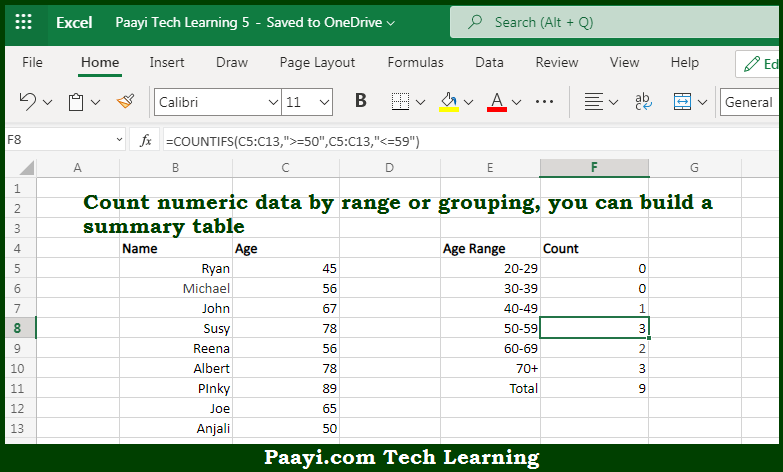# Learn How to Count Number By Range With COUNTIFS in Microsoft Excel

Written by | 0 Comments | 576 Views

In this article, you will learn how to COUNT various things in Mircosoft Excel using a single or combination of functions and its purpose in Microsoft Excel. You will also get to know how to Count Number By Range With COUNTIFS and see the generic formula.

Count Number By Range With COUNTIFS in Microsoft Excel

The main purpose of this formula is to count numeric data by range or grouping. Here we will learn how to count numbers by range with COUNTIFS in Microsoft Excel. That implies, with the help of a formula based on the COUNTIFS function you can able to count numeric data by range or grouping, you can even build a summary table to count values at each threshold. So, with the help of this formula, you can able to count numbers by range with COUNTIFS.

General Formula to Count Number By Range With COUNTIFS

=COUNTIFS(range,">=low",range,"<=high")

The Explanation for the Count Number By Range With COUNTIFSSo we know that with the help of the given formula above you can able to count numeric data by range or grouping. Here we will learn how to count numbers by range with COUNTIFS in Microsoft Excel. As we know that, The COUNTIFS function allows you to count values that meet multiple criteria with an AND relationship that is all criteria must be true. In the example provided here, we want to group the data by age range into 10-year brackets, so we use COUNTIFS. So, with the help of this formula, you can able to count numeric data by range or grouping.